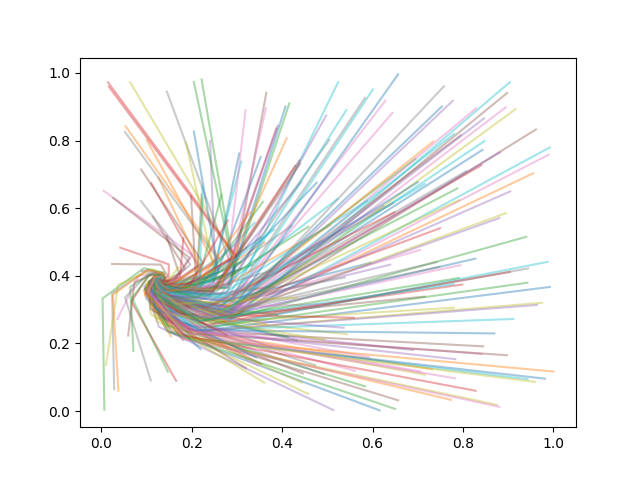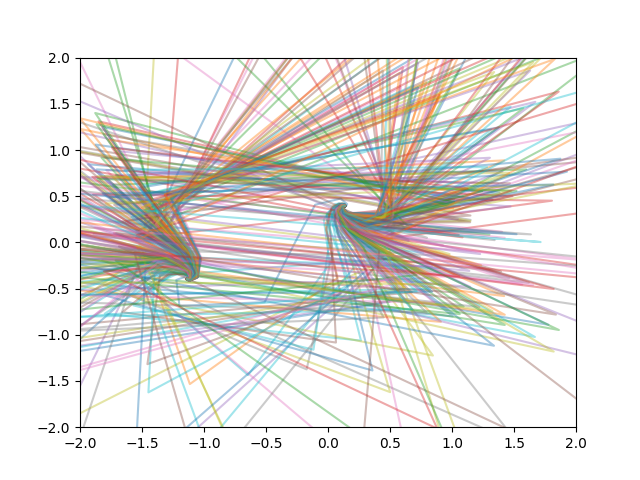# Fixed points of bilinear transformations

## Introduction

I was puzzled the first time I saw bilinear transformations, also known as Möbius transformations. I was in a class where everything had been abstract and general, and suddenly thing got very concrete and specific. I wondered why we had changed gears, and I wondered how there could be much to say about something so simple.

A bilinear transformation f has the formThe answer to my questions, which I did not realize at the time, was that bilinear transformations come up very often in applications, if not directly then indirectly as useful tools. For example, the electrical engineer’s Smith chart is a bilinear transformation. Also, most of the mental math tricks given here amount to bilinear approximations. And the Blaschke factors I wrote about yesterday are bilinear transformations.

## Fixed points

For this post I want to look at fixed points of bilinear transformations, solutions to f(x) = x where f is as above. This amounts to solving a quadratic equation.

The locations of the fixed points arewhereThe trace of the transformation is a + d, and the trace classifies the transformation into one of three categories: parabolic, elliptic, or loxodromic.

The trace could be any number in the complex plane, and all values of the trace correspond to the loxodromic case except when the trace is a real number in the interval [-2, 2].

In the loxodromic case there are two distinct fixed points: one attractive and one repulsive.

## Example

In this example I chose a = 1, b = i, c = 2, and d = 3. The two fixed points are at 0.136 + 0.393i and -1.136 – 0.393i.

I chose 200 starting points at random in the unit square and iterated the binlinear function. This produced the following graph.So apparently 0.136 + 0.393i is the attracting fixes point.

Next I started at 200 random starting points in a tight neighborhood of -1.136 – 0.393i., all within 0.00001 of the fixed point. This produced the following graph.If you start exactly on the repelling fixed point, you’ll stay there forever. But if you start a tiny distance away from this point you’ll end up at the attracting fixed point.